# How to Teach Halving Numbers

How to Teach Halving Numbers• To share an amount in half, separate the amount into two separate groups.
• Add one to each group one by one until there are no more items left.
• We can use the saying, “One for you, one for me” to share an amount in half.
• To teach the concept of halving a number, physical counters can be used.
• Check that the number of objects in each group is exactly the same.
• Here is the example of finding one half of 8.
• We move one counter into each group until all of the 8 counters have been moved.
• There are 4 counters in each group, so 4 is half of 8.
Share an amount into two separate groups to find half of it.

Use the phrase “One for me, one for you” to help you.• Here we will find half of ten.
• We count out ten counters.
• We separate the counters in half by making sure that there are the same number of counters on each side.
• There are 5 counters in each of the two groups.
• 5 is half of 10.Supporting Lessons

# Teaching the Concept of Halving

## How to Find One Half of a Number

To find one half of a number, divide it by 2. For larger numbers, split them into their tens and ones, halve these separately and then add them together.

For example, to find one half of 8, we divide it by 2.

Dividing by 2 means to split the total amount into two equal groups. There will be an equal number in each of the two groups.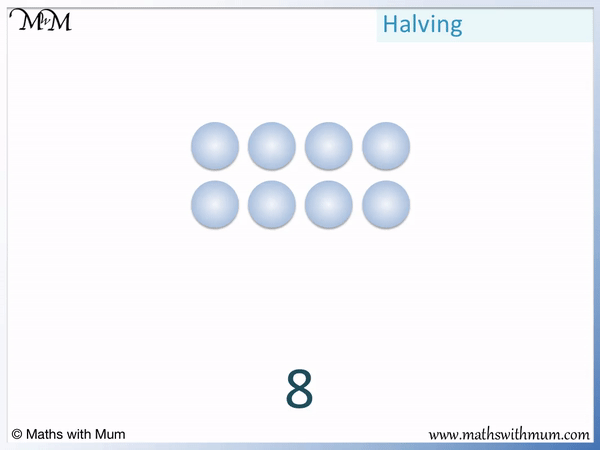We started with 8 counters.

We can see that there are 4 counters in each of the two groups and so, 4 is half of 8.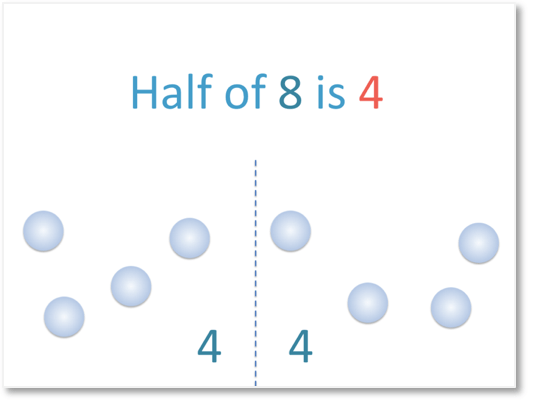We say that half of 8 is 4.

We can also say that 8 divided by 2 is 4.

We can write this as 8 ÷ 2 = 4.

## How to Teach the Concept of One Half

To teach the concept of one half, use physical items that can be divided easily into two, such as food items or piles of counters. Separate the amount into two equal groups by moving one item at a time. You can also use the phrase, “One for me, one for you” to share an amount in half.

To have one half of an amount, the amount must be split into two groups and there must be the same amount in each of the two groups.

When teaching halving, it is useful to have physical objects that can be used to compare the two groups.

When first identifying one half, objects such as fruit and vegetables can be easily cut into two equal parts. It helps to identify halves when they arise in daily life and show that the two halves are identical and that there are always two parts.

The key point to make when teaching the concept of one half, is that there are always two parts that must be exactly the same size.

## Teaching Halving Numbers

To teach the halving of smaller numbers, count out the required amount of counters and move them one by one into two equal piles. For larger numbers, break them down into tens and ones, halve each separately and then add up the result. It may help to write this down.

Start with introducing the idea of halving small numbers. To avoid confusion, only halve even numbers when first introducing the concept of halving. This is because halving odd numbers will result in fractions and decimals which are a more advanced concept that your child may not have understood just yet.

Here are the even numbers. Even numbers are numbers that can be halved exactly without remainder.Even numbers only end in 0, 2, 4, 6 or 8. When first teaching halving, it is best to pick a number ending in 0, 2, 4, 6 or 8 as it can be halved exactly.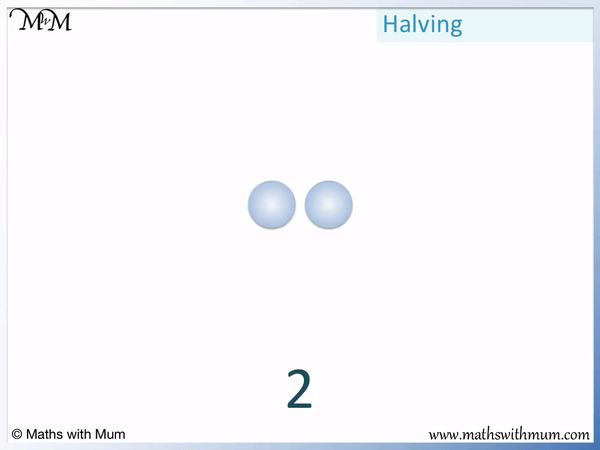Ask your child to move 1 counter to each side to introduce the idea of forming two equally sized groups.

Then move on to 4 counters.To help your child share an amount in half, use the phrase, “One for me, one for you”.

They should say, “One for me” as they place a counter in the first pile and then say, “One for you” as they place the counter in the other pile.

We can see that half of 4 is 2. Show them that there is exactly 2 counters in each pile. It is important to show that each pile must be the same size if an amount has been halved.

Here is one half of 6.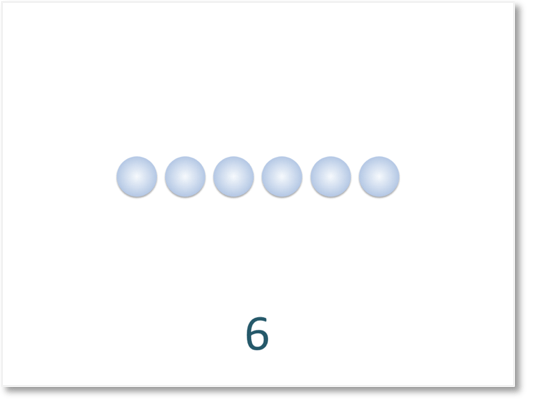One half of 6 is 3. Again, count the counters one at a time into two equal groups.

One half of 8 is 4.One half of 10 is 5.To halve larger numbers, separate the number into its tens and ones, halve these separately and then add the result together.

Use the following results to halve any larger number:

• One half of 2 is 1.
• One half of 4 is 2.
• One half of 6 is 3.
• One half of 8 is 4.
• One half of 10 is 5.

When teaching halving larger numbers, it is worth learning how to halve each of the even numbers to 10 off by heart. Use these to half the tens and ones parts of larger numbers.

For example, we will find one half of 16.

16 can be partitioned into 10 + 6.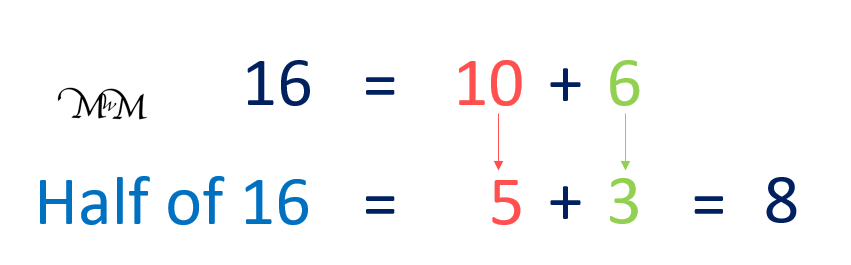From the list above, we know that half of 10 is 5 and half of 6 is 3.

We simply add 5 and 3 to make 8.

One half of 16 is 8.

In this example, we will find one half of 28.

We can write 28 as 10 + 10 + 8. We then halve each of these values and add up the result at the end.Half of 10 is 5, half of 10 is 5 and half of 8 is 4.

5 + 5 + 4 = 14 and so, half of 28 is 14.Now try our lesson on Multiplication is the Inverse of Division where we learn the relationship between multiplication and division.